## RS Aggarwal Class 9 Solutions Chapter 6 Coordinate Geometry Ex 6A

These Solutions are part of RS Aggarwal Solutions Class 9. Here we have given RS Aggarwal Class 9 Solutions Chapter 6 Coordinate Geometry Ex 6A.

Question 1.
Solution:
Co-ordinates of
A are ( – 6, 5)
B are (5, 4)
C are ( – 3, 2)
D are (2, – 2)
E are ( – 1, – 4)
Ans.Question 2.
Solution:
The given points have been plotted as shown in the adjoining graph.
Where X’OX and YOY’ are the axis: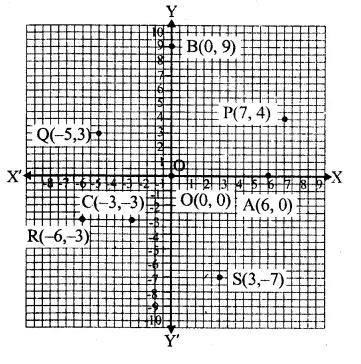Question 3.
Solution:
(i) (7, 0) lies on x-axis as its ordinate is (0)
(ii) (0, – 5) lies on y-axis as its abscissa is (0)
(iii) (0, 1) lies on y-axis as its abscissa is (0)
(iv) ( – 4, 0) lies on jc-axis as its ordinate is (0)
Ans.

Question 4.
Solution:
(i) ( – 6, 5) lies in second quadrant because A is of the type (-, +)
(ii) ( – 3, – 2) lies in third quadrant because A is of the type (-, -)
(iii) (2, – 9) lies in fourth quadrant because it is of the type (+, -).

Question 5.
Solution:
In the given equation, .
y = x+1
Put x = 0, then y = 0 + 1 = 1
x = 1, then, y = 1 + 1=2
x = 2, then, y = 2 + 1 = 3
Now, plot the points as given in the table given below on the graph, and join them as shown.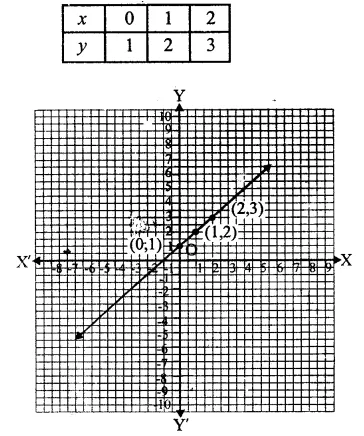Question 6.
Solution:
In the given equation
y = 3x + 2
Put x = 0,
then y = 3 x 0 + 2 = 0 + 2 = 2
x = 1, then
y = 3 x 1 + 2 = 3 + 2 = 5
and x = – 2, then
y = 3 x ( – 2) + 2 = – 6 + 2 = – 4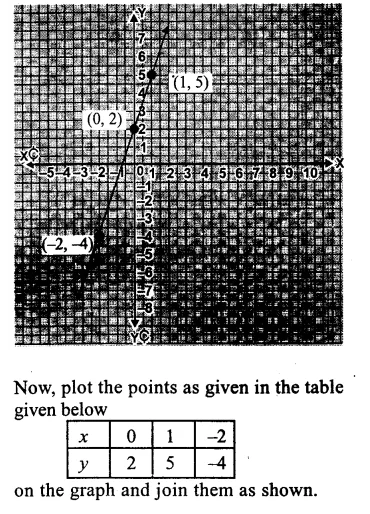Question 7.
Solution:
In the given equation,
y = 5x – 3
Put x = 1,y = 5 x 1 – 3 = 5 – 3 = 2
x = 0 then,
y = 5 x 0 – 3 = 0 – 3 = – 3
and x = 2, then
y = 5 x 2 – 3 = 10 – 3 = 7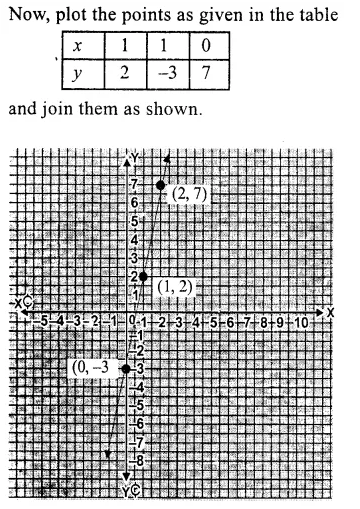Question 8.
Solution:
In the given equation,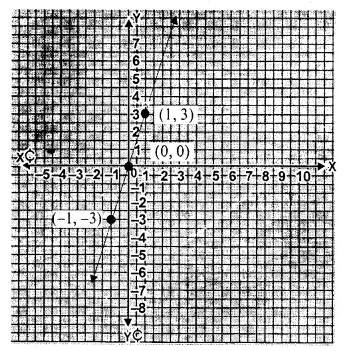y = 3x,
Put x = 0,
then y = 3 x 0 = 0
Put x = 1, then
y = 3 x 1 = 3
Put x = – 1, then
y = 3 ( – 1) = – 3
Now, plot the points as given in the table below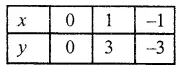and join them as shown.

Question 9.
Solution:
In the given equation, y = – x,
Put x = 1,
then y = – 1
Put x = 2, then
y = – 2
Put x = – 2, then
y = – ( – 2) = 2Hope given RS Aggarwal Class 9 Solutions Chapter 6 Coordinate Geometry Ex 6A are helpful to complete your math homework.

If you have any doubts, please comment below. Learn Insta try to provide online math tutoring for you.